Tamilnadu State Board New Syllabus Samacheer Kalvi 11th Chemistry Guide Pdf Chapter 4 Hydrogen Text Book Back Questions and Answers, Notes.

## Tamilnadu Samacheer Kalvi 11th Chemistry Solutions Chapter 4 Hydrogen

### 11th Chemistry Guide Hydrogen Text Book Back Questions and Answers

Textual Questions:

Question 1.
Which of the following statements about hydrogen is incorrect?
(a) Hydrogen ion, H3O+ exists freely in solution.
(b) Dihydrogen acts as a reducing agent.
(c) Hydrogen has three isotopes of which tritium is the most common.
(d) Hydrogen never acts as cation in ionic salts.
(c) Hydrogen has three isotopes of which tritium is the most common.

Question 2.
Water gas is
(a) H2O(g)
(b) CO + H2O
(c) CO + H2
(d) CO + N2
(c) CO + H2

Question 3.
Which one of the following statements is incorrect with regard to ortho and para dihydrogen ?
(a) They are nuclear spin isomers
(b) Ortho isomer has zero nuclear spin whereas the para isomer has one nuclear spin
(c) The para isomer is favoured at low temperatures
(d) The thermal conductivity of the para isomer is 50% greater than that of the ortho isomer.
(b) Ortho isomer has zero nuclear spin whereas the para isomer has one nuclear spin

Question 4.
Ionic hydrides are formed by
(a) halogens
(b) chalogens
(c) inert gases
(d) group one elements
(d) group one elements

Question 5.
Tritium nucleus contains
(a) 1p + 0n
(b) 2p + 1n
(c) 1p + 2n
(d) none of these
(c) 1p + 2nQuestion 6.
Non-stoichiometric hydrides are formed by
(b) carbon, nickel
(c) manganese, lithium
(d) nitrogen, chlorine
(b) carbon, nickel

Question 7.
Assertion:
Permanent hardness of water is removed by treatment with washing soda.
Reason:
Washing soda reacts with soluble calcium and magnesium chlorides and sulphates in hard water to form insoluble carbonates
(a) Both assertion and reason are true and reason is the correct explanation of assertion.
(b) Both assertion and reason are true but reason is not the correct explanation of assertion.
(c) Assertion is true but reason is false
(d) Both assertion and reason are false
(a) Both assertion and reason are true and reason is the correct explanation of assertion.

Question 8.
If a body of a fish contains 1.2 g hydrogen in its total body mass, if all the hydrogen is replaced with deuterium then the increase in body weight of the fish will be
(a) 1.2 g
(b) 2.4 g
(c) 3.6 g
(d) $$\sqrt{4.8}$$ g
(a) 1.2 g

Question 9.
The hardness of water can be determined by volumetrically using the reagent
(a) sodium thio sulphate
(b) potassium permanganate
(c) hydrogen peroxide
(d) EDTA
(d) EDTA

Question 10.
The cause of permanent hardness of water is due to
(a) Ca(HCO3)2
(b) Mg(HCO3)2
(c) CaCl2
(d) MgCO3
(c) CaCl2Question 11.
Zeolite used to soften hardness of water is, hydrated
(a) Sodium aluminium silicate
(b) Calcium aluminium silicate
(c) Zinc aluminium borate
(d) Lithium aluminium hydride
(a) Sodium aluminium silicate

Question 12.
A commercial sample of hydrogen peroxide marked as 100 volume H2O2, it means that
(a) 1 ml of H2O2 will give 100 ml O2 at STP
(b) 1 L of H2O2 will give 100 ml O2 at STP
(c) 1 L of H2O2 will give 22.4 L O2
(d) 1 ml of H2O2 will give 1 mole of O2 at STP
(a) 1 ml of H2O2 will give 100 ml O2 at STP

Question 13.
When hydrogen peroxide is shaken with an acidified solution of potassium dichromate in presence of ether, the ethereal layer turns blue due to the formation of
(a) Cr2O3
(b) CrO42-
(c) CrO(O2)2
(d) none of these
(c) CrO(O2)2

Question 14.
For decolourisation of 1mole of acidified KMnO4, the moles of H2O2 required is
(a) $$\frac{1}{2}$$

(b) $$\frac{3}{2}$$

(c) $$\frac{5}{2}$$

(d) $$\frac{7}{2}$$
(c) $$\frac{5}{2}$$

Question 15.
Volume strength of 1.5 NH2O2 is
(a) 1.5
(b) 4.5
(c) 16.8
(d) 8.4
(d) 8.4Question 16.
The hybridisation of oxygen atom is H2O and H2O2 are, respectively
(a) sp and sp3
(b) sp and sp
(c) sp and sp2
(d) sp3 and sp3
(d) sp3 and sp3

Question 17.
The reaction H3PO2 + D2O → H2DPO2 + HDO indicates that hypo-phosphorus acid is
(a) tribasic acid
(b) dibasic acid
(c) mono basic acid
(d) none of these
(c) mono basic acid
Solution:
Hypophosphorus acid on reaction with D2O, only one hydrogen is replaced by deuterium and hence it is mono basic.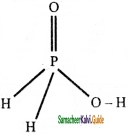Question 18.
In solid ice, oxygen atom is surrounded
(a) tetrahedrally by 4 hydrogen atoms
(b) octahedrally by 2 oxygen and 4 hydrogen atoms
(c) tetrahedrally by 2 hydrogen and 2 oxygen atoms
(d) octahedrally by 6 hydrogen atoms
(a) tetrahedrally by 4 hydrogen atoms
Solution: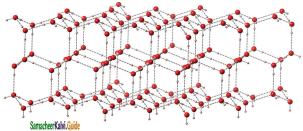Question 19.
The type of H-bonding present in ortho nitro phenol and p-nitro phenol are respectively
(a) inter molecular H-bonding and intra molecular f H-bonding
(b) intra molecular H-bonding and inter molecular H-bonding
(c) intra molecular H – bonding and no H – bonding
(d) intra molecular H -bonding and intra molecular H-bonding
(b) intra molecular H-bonding and inter molecular H-bonding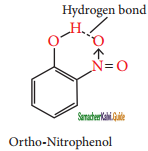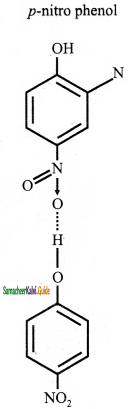Question 20.
Heavy water is used as
(a) modulator in nuclear reactions
(b) coolant in nuclear reactions
(c) both (a) and (b)
(d) none of these
(c) both (a) and (b)
Solution:
(c) Heavy water is used as moderator as well as coolant in nuclear reactions.

Question 21.
Water is a
(a) basic oxide
(b) acidic oxide
(c) amphoteric oxide
(d) none of these
(c) amphoteric oxide
Solution:
Water is a amphoteric oxide.II. Write brief answer to the following questions:

Question 22.
Explain why hydrogen is not placed with the halogen in the periodic table.
The electron affinity of hydrogen is much less than that of halogen atoms. Hence, the tendency of hydrogen to form hydride ion is low compared to that of halide ions. In most of its compounds hydrogen exists in +1 oxidation state. Therefore, it is reasonable to place the hydrogen in group -1 along with alkali metals and not placed with halogens.

Question 23.
A cube at the 0°C is placed in some liquid water at 0°C, the ice cube sinks – Why?
In ice, each atom is surrounded tetrahedrally by four water molecules through hydrogen bonds. That is, the presence of two hydrogen atoms and two lone pairs of electron on oxygen atoms in each water molecule allows formation of a three-dimensional structure.

This arrangement creates an open structure, which accounts for the lower density of ice compared with water at 0°C. While in liquid water, unlike ice where hydrogen bonding occurs over a long-range, the strong hydrogen bonding prevails only in a short range and therefore the denser packing. Hence, ice cube sinks in water.

Question 24.
Discuss the three types of Covalent hydrides.
Covalent hydrides are the compound in which hydrogen is attached to another element by sharing of electrons. The most common examples of covalent hydrides of non-metals are methane, ammonia, water and hydrogen chloride. Covalent hydrides are further divided into three categories, viz., electron
precise (CH4 C2H6), electron deficient (B2H6) and electron-rich hydrides (NH3H2 O). Since most of the covalent hydrides consists of discrete, small molecules that have relatively weak intermolecular forces, they are generally gases or volatile liquids.

Question 25.
Predict which of the following hydrides is a gas on a solid (a) HCl (b) NaH. Give your reason.
Sodium hydride (NaH) is a gas on a soiid. NaH is prepared by direct reaction of hydrogen gas with liquid sodium and it has NaCl crystal structure. It is a tendency of deprotonating in many organic reactions. NaH is used in fuel cells.Question 26.
Write the expected formulas for the hydrides of 4th period elements. What is the trend in the formulas? In what way the first two numbers of the series different from the others?
The expected formulas of the hydrides of 4th period elements are MH or MH2. However, except the first two members, many of the elements form non- stoichiometric interstitial hydrides with variable composition. The first two members of the period alkali metal (Potassium) and alkali earth metal (Calcium) forms ionic hydrides.

Question 27.
Write chemical equation for the following reactions.
(i) reaction of hydrogen with tungsten (VI) oxide NO3 on heating.
(ii) hydrogen gas and chlorine gas.
(i) Hydrogen can be used to reduce metal oxide into metal. Hydrogen reduces tungsten(VI) oxide into tungsten.
WO3 + 3H2 → W + 3H2O

(ii) Hydrogen reacts with chlorine at room temperature under light gives hydrogen chloride.
H2(g) + Cl2(g) → 2HCl(g)

Question 28.
Complete the following chemical reactions and classify them into (a) hydrolysis (b) redox (c) hydration reactions.
(i) KMnO4 + H2O2
(ii) CrCl3 + H2O →
(iii) CaO + H2O →
(i) KMnO4 + H2O2 → 2KMnO2 + 2KOH + 2H2O + 3O2
The reaction of potassium permanganate with hydrogen peroxide is a redox reaction.

(ii) CrCl3 + H2O → [Cr(H2O)6]Cl3
It is a hydration reaction. Many salts crystallized from aqueous solutions form hydrated crystals. The water in the hydrated salt may form co-ordinate bonds.

(iii) CaO + H2O → Ca(OH)2
It is a hydrolysis reaction. Calcium oxide hydrolyses to calcium hydroxide.

Question 29.
Hydrogen peroxide can function as an oxidising agent as well as reducing agent. Substantiate this statement with suitable examples.
Hydrogen peroxide can act both as an oxidizing agent and a reducing agent. Oxidation is usually performed in acidic medium while the reduction reactions are performed in basic medium.

In acidic conditions:
H2O2 + 2H+ + 2e → 2H2O (E° = +1.77 V)
For example
2FeSO4 + H2SO4 + H2O2 → Fe2(SO4)3 + 2H2O

In basic conditions:
HO2 + OH → O2 + H2O + 2e (E° = + 0.08V)

For Example,
2 KMnO4(aq) + 3H2O2(aq) → 2MnO2 + 2KOH + 2H2O + 3O2(g)

Question 30.
Do you think that heavy water can be used for drinking purposes?
Heavy water cannot be used for drinking purposes because it does not form hydrogen bonding.Question 31.
What is water-gas shift reaction?
The carbon monoxide of the water gas can be converted to carbon dioxide by mixing the gas mixture with more steam at 400°C and passed over a shift converter containing iron/copper catalyst. This reaction is called as water-gas shift reaction.
CO + H2O → CO2 + H2
The CO2 formed in the above process is absorbed in a solution of potassium carbonate.
CO2 + K2CO3 + H2O → 2KHCO3

Question 32.
Justify the position of hydrogen in the periodic table?
The hydrogen has the electronic configuration of 1s1 which resembles with ns1 general valence shell configuration of alkali metals and shows similarity with them as follows:
1. It forms unipositive ion (H+) like alkali metals {Na+, K+, Cs+)
2. It forms halides (HX), oxides, (H2O), peroxides (H2O2) and sulphides (H2S) like alkali metals (NaX, Na2O, NaH2OH2, NaH2S)
3. It also acts as a reducing agent.

However, unlike alkali metals which have ionization energy ranging from 377 to 520 kJ mol-1, the hydrogen has 1,314 kJ mol-1 which is much higher than alkali metals.

Like the formation of halides (X) from halogens, hydrogen also has a tendency to gain one electron to form hydride ion (H) whose electronic configuration is similar to the noble gas, helium. However, the electron affinity of hydrogen is much less than that of halogen atoms. Hence, the tendency of hydrogen to form hydride ion is low compared to that of halogens to form the halide ions as evident from the following reactions:
$$\frac{1}{2}$$ H2 + e → H                         ∆H = +36 kcalmol-1
$$\frac{1}{2}$$ Br2 + e → Br                       ∆H = -55 kcalmol-1

Since, hydrogen has similarities with alkali metals as well as the halogens; it is difficult to find the right position in the periodic table. However, in most of its compounds hydrogen exists in +1 oxidation state. Therefore, it is reasonable to place the hydrogen in group 1 along with alkali metals as shown in the latest periodic table published by IUPAC.

Question 33.
What are isotopes? Write the names of isotopes of hydrogen.
Atoms of the same element having same atomic number and different mass number are called isotopes.
Hydrogen has three naturally occurring isotopes, viz., protium (1H1 or H), deuterium (1H2 or D) and tritium (1H3 or T). Protium 1H1 is the predominant form (99.985 %) and it is the only isotope that does not contain a neutron. Deuterium, also known as heavy hydrogen, constitutes about 0.015 %. The third isotope, tritium is a radioactive isotope of hydrogen which occurs only in traces (~1 atom per 1018 hydrogen atoms). Due to the existence of these isotopes naturally occurring hydrogen exists as H2, HD, D2, HT, T2, and DT.

Question 34.
Give the uses of heavy water.
The uses of heavy water are as follows
(i) Heavy water is widely used as moderator in nuclear reactors as it can lower the energies of fast neutrons
(ii) It is commonly used as a tracer to study organic reaction mechanisms and mechanism of metabolic reactions
(iii) It is also used as a coolant in nuclear reactors as it absorbs the heat generated.

Question 35.
Explain the exchange reactions of deuterium.
When compounds containing hydrogen are treated with D2O, hydrogen undergoes an exchange for deuterium. This reaction is known as exchange reaction of deuterium.
2NaOH + D2O → 2NaOD + HOD

HCl + D2O → DCl + HOD

NH4Cl + 4D2O → ND4Cl + 4HOD

These exchange reactions are useful in determining the number of ionic hydrogens present in a given compound. For example, when D2O is treated with of hypo-phosphorus acid only one hydrogen atom is exchanged with deuterium. It indicates that, it is a monobasic acid.
H3PO2 + D2O → H2DPO2 + HDO
It is also used to prepare some deuterium compounds:

Al4C3 + 12D2O → 4Al(OD)3 + 3CD4
CaC2 + 2D2O → Ca(OD)2 + C2D2
Mg3N2 + 6D2O → 3Mg(OD)2 + 2ND3
Ca3P2 + 6D2O → 3Ca(OD)2 + 2PD3Question 36.
How do you convert parahydrogen into ortho hydrogen?
At room temperature, normal hydrogen consists of about 75% ortho-form and 25% para-form. As the ortho-form is more stable than para-form, the conversion of one isomer into the other is a slow process. However, the equilibrium shifts in favour of para hydrogen when the temperature is lowered.

The para-form can be catalytically transformed into I ortho-form using platinum or iron. Alternatively, it can also be converted by passing an electric j discharge, heating above 800°C and mixing with paramagnetic molecules such as O2, NO, NO2 or with nascent/atomic hydrogen.

Question 37.
Mention the uses of deuterium.
(i) Deuterium is used to prepare heavy water which is used as moderator in nuclear reactors.
(ii) Deuterium exchange reactions are useful in determining the number of ionic hydrogens present in a given compound.
(iii) It is also used to prepare some deuterium compounds.

Question 38.
Explain preparation of hydrogen using electrolysis.
High purity hydrogen (> 99.9 %) is obtained by the electrolysis of water containing traces of acid or alkali or the electrolysis of aqueous solution of sodium hydroxide or potassium hydroxide using a nickel anode and iron cathode. However, this process is not economical for large-scale production.
At anode:
2OH → H2O + $$\frac{1}{2}$$O2 + 2e
At cathode:
2H2O + 2e → 2OH + H2
Overall reaction:
H2O → H2 + $$\frac{1}{2}$$O2

Question 39.
A group-1 metal (A) which is present ¡n common salt reacts with (B) to give compound (C) in which hydrogen is present in -1 oxidation state. (B) on reaction with a gas to give universal solvent (D). The compound (D) on reacts with (A) to give (B), a strong base. Identify A, B, C, D and E. Explain the reactions.
The metal belongs to Group-1 which is present in common salt is Sodium(A).
The metal reacts with (B) to give compound (C) in which hydrogen is present in -1 oxidation state.
2Na + H2 → 2NaH
Hence, the (B) is hydrogen and (C) is sodium hydride. (B) on reaction with a gas to give universal solvent (D).
H2 + O2 → 2NaOH
The universal solvent (D) is water. The compound (D) on reaction with (A) to give (E) which is a strong base.
H2O + 2Na → 2NaOH
The Compound (E) is Sodium hydroxide.
A – Sodium (Na)
B – Hydrogen (H2)
C – Sodium Hydride (NaH)
D – Water (H2O)
E – Sodium hydroxide (NaOH)

Question 40.
An isotope of hydrogen (A) reacts with diatomic molecule of element which occupies group number 16 and period number 2 to give compound (B) is used as a moderatorin nuclear reaction. (A) adds on to a compound (C), which has the molecular formula C3H6 to give (D). Identify A, B, C and D.
An isotope of hydrogen (A) reacts with diatomic molecule of element which occupies group number 16 and period number 2 to give compound (B) is used as a moderator in nuclear reaction.
2D2 + O2 → 2D2O
The isotope of hydrogen is deuterium(A), diatomic element is oxygen and the compound (B) is heavy water.
(A) adds on to a compound (C), which has the molecular formula C3H6 to give (D).
CH3 – CH = CH2 + D2 → CH3 – CHD – CH2D
(C)                                     (D)
The compound (C) is propene and (D) is 1, 2 deutropropane.
A – Deuterium (D2)
B – Heavy water (D2O)
C – Propene (CH3 – CH = CH2)
D – 1, 2 deutero propene (CH3 – CHD – CH2D)Question 41.
NH3 has exceptionally high melting point and boiling point as compared to those of the hydrides of the remaining element of group 15- Explain.
When a hydrogen atom is covalently bonded to a highly electronegative atom such as nitrogen, the bond is polarized. Due to this effect, the polarized hydrogen atom is able to form a weak electrostatic interaction with another electronegative atom present in the vicinity.

This interaction is called hydrogen bonding. Hence, NH3 has exceptionally high melting point and boiling point as compared to those of the hydrides of the remaining element of group 15 due to intermolecular hydrogen bonding.

Question 42.
Why interstitial hydrides have a lower density than the parent metal?
In interstitial hydrides, hydrogen occupies the interstitial sites. These hydrides show properties similar to parent metals. Most of these hydrides are non-stoichiometric with variable composition. Hence, interstitial hydrides have lower density than the parent metal.

Question 43.
How do you expect the metallic hydrides to be useful for hydrogen storage?
Metallic hydrides are usually obtained by hydrogenation of metals and alloys in which hydrogen occupies the interstitial sites (voids). Most of the hydrides are non-stoichiometric with variable composition (TiH 1.5 – 1.8 and PdH0.6 – 0.8), some are relatively light, inexpensive and thermally unstable which make them useful for hydrogen storage applications.

Question 44.
Arrange NH3, H2O and HF in the order of increasing magnitude of hydrogen bonding and explain the basis for your arrangement.
When a hydrogen atom is covalently bonded to a highly electronegative atom such as nitrogen, the bond is polarized. Due to this effect, the polarized hydrogen atom is able to form a weak electrostatic interaction with another electronegative atom present in the vicinity. This interaction is called hydrogen bonding. The magnitude of hydrogen bonding increases with the increase in electronegativity of the atom. Hence, the increasing magnitude of hydrogen bonding of NH3, H2O and HF follows the order NH3 < H2O < HF.

Question 45.
Compare the structures of HO and HO.
Both in gas-phase and liquid-phase, the molecule adopts a skew conformation due to repulsive interaction of the OH bonds with lone-pairs of electrons on each oxygen atom. Indeed, it is the smallest molecule known to show hindered rotation about a single bond.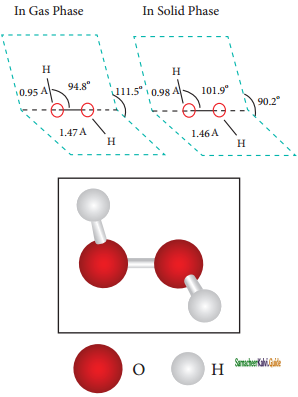H2O2 has a non-polar structure. The molecular dimensions in the gas phase and solid phase differ as shown in figure. Structurally, H2O2 is represented by the dihydroxyl formula in which the two OH-groups do not lie in the same plane.

One way of explaining the shape of hydrogen peroxide is that the hydrogen atoms would lie on the pages of a partly opened book, and the oxygen atoms along the spine. In the solid phase of molecule, the dihedral angle reduces to 90.2° due to hydrogen bonding and the O-O-H angle expands from 94.8° to 101.9°. Water has bent structure and the H-O-H bond angle is 104.5°.Question 1.
A simplest atom which contains one electron and one proton is
(a) Helium
(b) Deuterium
(c) Hydrogen
(d) Tritium
(c) Hydrogen

Question 2.
Hydrogen has similarities with
(a) Alkali metals and halogens
(b) Alkaline earth metals and halogens
(c) Alkalimetals and noble gases
(d) Halogens and noble gases.
(a) Alkali metals and halogens

Question 3.
Which of the following properties of hydrogen similar to alkali metals?
1. It forms uni negative ion.
2. It forms halides, oxides and sulphides similar to alkali metals.
3. It also acts as a reducing agent.
(a) 1 and 2
(b) 2 and 3
(c) 1 and 3
(d) 1,2 and 3
(b) 2 and 3

Question 4.
Ionisation energy of hydrogen is (in kJ mol-1)
(a) 377
(b) 520
(c) 1413
(d) 1314
(d) 1314

Question 5.
Hydrogen is placed in the ________ of the periodic table.
(a) group – 1
(b) group – 17
(c) group – 18
(d) group – 2
(a) group – 1Question 6.
The predominant isotope of hydrogen is
(a) deuterium
(b) protium
(c) tritium
(d) heavy hydrogen
(b) protium

Question 7.
The radioactive isotope of hydrogen is
(a) protium
(b) deuterium
(c) tritium
(d) heavy hydrogen
(c) tritium

Question 8.
The number of naturally occurring hydrogens due to existence of isotopes is
(a) 6
(b) 5
(c) 4
(d) 3
(a) 6

Question 9.
The half life period of tritium is ______(in years).
(a) 13.2
(b) 10.5
(c) 12.3
(d) 15.8
(c) 12.3

Question 10.
The correct order of melting point of isotopes of hydrogen is
(a) H < D < T
(b) T < D < H
(c) H < T < D
(d) D < H < T
(a) H < D < TQuestion 11.
The percentage of ortho and para forms of normal hydrogen at room temperature is
(a) 25% and 75%
(b) 40% and 60%
(c) 60% and 40%
(d) 75% and 25%
(d) 75% and 25%

Question 12.
Which of the following statement is correct about ortho-para hydrogen?
(a) The magnetic moment of para hydrogen is twice that of a proton.
(b) Ortho form is more stable than para form
(c) Ortho form can be catalytically converted into para form using platinum.
(d) At room temperature, normal hydrogen consists of 75% para form.
(b) Ortho form is more stable than para form

Question 13.
The composition of syngas is
(a) CO + N2
(b) CO + H2O
(c) CO + H2
(d) CO2 + H2
(c) CO + H2

Question 14.
During electrolysis of water containing traces of acid hydrogen is liberated at
(a) cathode
(b) anode
(c) both anode and cathode
(d) none of the above
(a) cathode

Question 15.
The conversion of carbon monoxide of the water gas into carbon dioxide is called water gas _______ reaction.
(a) displacement
(b) decomposition
(c) shift
(d) conversion
(c) shiftQuestion 16.
The catalyst used in the water gas shift reaction is
(a) Copper
(b) Nickel
(c) Platinum
(a) Copper

Question 17.
The CO2 formed in the water gas shift reaction is absorbed in a solution of
(a) Potassium bicarbonate
(b) Sodium chloride
(c) Potassium sulphate
(d) Potassium carbonate
(d) Potassium carbonate

Question 18.
The percentage of heavy water in normal water is
(a) 1.6 × 104
(b) 1.6 × 10-4
(c) 1.6 × 10-3
(d) 1.6 × 102
(b) 1.6 × 10-4

Question 19.
When water is completely electrolysed, the gas liberated is/are
(a) H2
(b) D2
(c) H2 and D2
(d) H2 and T2
(c) H2 and D2

Question 20.
Lithium Aluminium Hydride is
(a) [LiAlH3]
(b) Li[Al2H4]
(c) Li[AlH2]
(d) Li[AlH4]
(d) Li[AlH4]Question 21.
Deuterium oxide is called
(a) hard water
(b) soft water
(c) heavy water
(d) heavy hydrogen
(c) heavy water

Question 22.
Ammonia is synthesized by ______ process.
(a) Haber’s
(b) Bergius
(c) Decon’s
(d) Solvay
(b) Bergius

Question 23.
Statement – I:
Tritium is a β-emitter.
Statement – II:
Radioactive decay of tritium gives $${ }_{2}^{3} \mathrm{He}$$ and $${ }_{1}^{0} e$$.
The correct statement/s is/are
(a) I alone
(b) II alone
(c) both I and II
(d) both are incorrect.
(c) both I and II

Question 24.
The high melting and boiling points of water is due to
(a) Covalent bonding
(b) Hydrogen bonding
(c) Ionic bonding
(d) co-ordinate bonding
(b) Hydrogen bonding

Question 25.
Chlorine reacts with water and forms _____ and _____ respectively.
(a) HCl and HOCl
(b) H2 and HCl
(c) HOCl and H2
(d) HCl and ClO2
(c) HOCl and H2Question 26.
Water is an ______ oxide.
(a) acidic
(b) basic
(c) amphoteric
(d) neutral
(a) acidic

Question 27.
Hydrolysis of P4O10 gives
(a) HPO2
(b) H4P2O7
(c) H3PO3
(d) H2PO4
(d) H2PO4

Question 28.
In CuSO4.5H2O, the number of water molecules form co-ordinate bonds is
(a) 4
(b) 5
(c) 3
(d) 1
(a) 4

Question 29.
Flourine reacts with water and liberates
(a) hydrogen
(b) oxygen
(c) Fluorine dioxide
(d) HOF
(b) oxygen

Question 30.
The number water molecules in hydrated crystal of Chromium chloride salt is
(a) 5
(b) 6
(c) 4
(d) 3
(b) 6Question 31.
The most common metal ions present in the hard water are
(a) Magnesium and Iron
(b) Calcium and Aluminium
(c) Magnesium and Calcium
(d) Manganese and Calcium
(c) Magnesium and Calcium

Question 32.
Temporary hardness of water is removed by ______ method.
(a) Dewar
(b) Clark’s
(c) Leibeg
(d) Haber
(b) Clark’s

Question 33.
Permanent hardness of water is due to the presence of soluble salts of _____ and ______ of magnesium and calcium.
(a) carbonates and bicarbonates
(b) chlorides and carbonates
(c) bicarbonates and sulphates
(d) chlorides and sulphates
(d) chlorides and sulphates

Question 34.
The ion exchange bed used for the softening of hard water is
(a) Borates
(b) Zeolites
(c) Fluorides
(d) Phosphates
(b) Zeolites

Question 35.
The general formula of zeolites is
(a) NaOAl2O3. xSiO2. yH2O
(b) Na2O.Al2O3. ySiO2. xH2O
(c) NaOH.Al2O3. xSiO2. yH2O
(d) NaO.Al (OH)3 .xSiO2. yH2O
(a) NaOAl2O3. xSiO2. yH2OQuestion 36.
________ reactions are useful in determining the number of ionic hydrogens present in a given compound.
(a) Oxygen exchange
(b) Metal exchange
(c) Deuterium exchange
(d) Deuterium decomposition
(c) Deuterium exchange

Question 37.
_______ is used as a moderator and coolant in nuclear reactors.
(a) Heavy hydrogen
(b) Ortho hydrogen
(c) Hydrogen peroxide
(d) Heavy water
(d) Heavy water

Question 38.
Autoxidation of 2-alkyl anthraquinol gives
(a) Hydrogen peroxide
(b) Heavy water
(c) Hydrogen
(d) Water
(a) Hydrogen peroxide

Question 39.
The percentage of hydrogen peroxide in ‘100 volume’ is
(a) 40
(b) 30
(c) 50
(d) 20
(b) 30

Question 40.
Hydrogen peroxide solutions are stored in ______ container
(a) glass
(b) alkali metal
(c) plastic
(d) wooden
(c) plasticQuestion 41.
______ present in the glass catalyses the disproportionation reaction of hydrogen peroxide.
(a) Silica
(b) Alkali metals
(c) fluorine
(d) Oxygen
(b) Alkali metals

Question 42.
Disproportionation of hydrogen peroxide gives
(a) oxygen and hydrogen
(b) hydrogen and water
(c) hydrogen and ozone
(d) oxygen and water
(d) oxygen and water

Question 43.
Which of the following statement/s are true about hydrogen peroxide?
1. It can act both as an oxidizing agent and a reducing agent.
2. It is used in water treatment to oxidize pollutants.
3. It is used as mild analgesic.
4. It restores the white colour of the old paintings,
(a) 1, 2 and 3
(b) 1, 3 and 4
(c) 1, 2 and 4
(d) 2, 3 and 4
(c) 1, 2 and 4

Question 44.
White pigment is
(a) Pb2(OH)2(C03)3
(b) Pb3(OH)2(C03)2
(c) Pb3(OH)(CO3)2
(d) Pb2(OH)(CO3)3
(b) Pb3(OH)2(C03)2

Question 45.
The smallest molecule which shows hindered rotation about single bond is
(a) Hydrogen peroxide
(b) Water
(c) Deuterium oxide
(d) hydrogen
(a) Hydrogen peroxideQuestion 46.
Compounds in which hydrogen is attached to another element by sharing of electrons are called _________ hydrides.
(a) Interstitial
(b) Molecular
(c) Saline
(d) Metallic
(b) Molecular

Question 47.
Which of the following molecule shows intramolecular hydrogen bond?
(a) Water
(b) Ammonia
(c) Salicylaldehyde
(d) Para-nitrophenol
(c) Salicylaldehyde

Question 48.
Each water molecule is linked to ______ other molecules through hydrogen bonds.
(a) five
(b) four
(c) six
(d) two
(b) four

Question 49.
Which one of the following is a covalent hydride?
(a) NH3
(b) BeH2
(c) NaH
(d) ZrH2
(a) NH3

Question 50.
Hypo-phosphorus is a ______ acid.
(a) dibasic
(b) tribasic
(c) monobasic
(d) tetrabasic
(c) monobasicII. Very Short Question and Answers (2 Marks):

Question 1.
What are the isotopes of hydrogen?
Hydrogen has three naturally occurring isotopes, viz., protium (1H1 or H), deuterium (1H2 or D) and tritium (1H3 or T).

Question 2.
Write the physical properties of Hydrogen?
Hydrogen is a colorless, odorless, tasteless, lightest and highly flammable gas. It is a non-polar diatomic molecule. It can be liquefied under low temperature and high pressure. Hydrogen is a good reducing agent

Question 3.
How is tritium prepared?
Tritium is artificially prepared by bombarding lithium with slow neutrons in a nuclear fission reactor. The nuclear transmutation reaction for this process is as follows.
$${ }_{3}^{6} L i$$ + $${ }_{0}^{1} n$$ → $${ }_{2}^{4} \mathrm{He}$$ + $${ }_{1}^{3} T$$

Question 4.
What are ortho and para hydrogens?
In the hydrogen atom, the nucleus has a spin. When molecular hydrogen is formed, the spins of two hydrogen nuclei can be in the same direction or in the opposite direction as shown in the figure. These two forms of hydrogen molecules are called ortho and para hydrogens respectively.

Question 5.
Write the different forms of naturally occurring hydrogen?
Due to the existence of three isotopes of hydrogen, Protium (H), Deuterium (D) and Tritium(T), naturally occurring hydrogen exists as H2, HD, D2, HT, T2, and DT.Question 6.
How will you convert para hydrogen into ortho hydrogen?
The para-form can be catalytically transformed into ortho-form using platinum or iron. Alternatively, it can also be converted by passing an electric discharge, heating above 800°C and mixing with paramagnetic molecules such as O2, NO, NO2 or within ascent/atomic hydrogen.

Question 7.
How is pure hydrogen prepared?
High purity hydrogen (> 99.9 %) is obtained by the electrolysis of water containing traces of acid or alkali or the electrolysis of aqueous solution of sodium hydroxide or potassium hydroxide using a nickel anode and iron cathode. However, this process is not economical for large-scale production.
At anode:
2OH → H2O + $$\frac{1}{2}$$O2 + 2e

At cathode:
2H2O + 2e → 2OH + H2

Overall reaction:
H2O → H2 + $$\frac{1}{2}$$O2

Question 8.
How is hydrogen prepared by steam reforming reaction?
Hydrogen is produced in large scale by steam-reforming of hydrocarbons. In this method, hydrocarbon such as methane is mixed with steam and passed over nickel catalyst in the range 800- 900°C and 35 atm pressure.
CH4 + H2O → CO + 3H2

Question 9.
What is water gas? How is it prepared?
The mixture of carbon monoxide and hydrogen is called water gas. When steam is passed over a red- hot coke to produce carbon monoxide and hydrogen. The mixture of gases produced in this way is known as water gas (CO + H2).
C + H2O → (CO + H2)Water gas /Syngas

Question 10.
What is syngas? Why is it called so?
Water gas is also called as syngas as it is used in the synthesis of organic compounds such as methanol and simple hydrocarbons.Question 11.
How is Deuterium prepared?
Normal water contains 1.6 × 10-4 percentage of heavy water. The dissociation of protium water (H2O) is more than heavy water (D2O). Therefore, when water is electrolysed, hydrogen is liberated much faster than D2. The electrolysis is continued until the resulting solution becomes enriched in heavy water. Further electrolysis of the heavy water gives deuterium.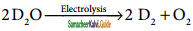Question 12.
How is Tritium prepared?
Tritium is present only in trace amounts. So it can be artificially prepared by bombarding lithium with slow neutrons in a nuclear fission reactor. The nuclear transmutation reaction for this process is as follows.
$${ }_{3}^{6} L i$$ + $${ }_{0}^{1} n$$ → $${ }_{2}^{4} \mathrm{He}$$ + $${ }_{1}^{3} T$$

Question 13.
Write the physical properties of hydrogen.
Hydrogen is a colorless, odorless, tasteless, lightest and highly flammable gas. It is a non-polar diatomic molecule. It can be liquefied under low temperature and high pressure. Hydrogen is a good reducing agent.

Question 14.
What is deuterium exchange reaction?
Deuterium can replace reversibly hydrogen in compounds either partially or completely depending upon the reaction conditions. These reactions occur in the presence of deuterium or heavy water.
CH4 + 2D2 → CD4 + 2H2
2NH3 + 3D2 → 2ND3 + 3H2

Question 15.
Write the physical properties of water.
Water is a colourless and volatile liquid. The peculiar properties of water in the condensed phases are due to the presence of inter molecular hydrogen bonding between water molecules. Hydrogen bonding is responsible for the high melting and boiling points of water.Question 16.
Water is an amphoteric oxide. Give reason.
Water is an amphoteric oxide. It has the ability to accept as well as donate protons and hence it can act as an acid or a base. For example, in the reaction with HC1 it accepts proton where as in the reaction with weak base ammonia it donates proton.
NH3 + H2O → NH4+ + OH
HCl + H2O → H3O+ + Cl

Question 17.
What is hard water? Give its types.
Hard water contains high amounts of mineral ions. The most common ions found in hard water are the soluble metal cations such as magnesium & calcium, though iron, aluminium, and manganese may also be found in certain areas. Presence of these metal salts in the form of bicarbonate, chloride and sulphate in water makes water ‘hard’.

Question 18.
What is meant by temporary hardness of water?
Temporary hardness is primarily due to the presence of soluble bicarbonates of magnesium and calcium.

Question 19.
What is permanent hardness of water?
Permanent hardness of water is due to the presence of soluble salts of magnesium and calcium in the form of chlorides and sulphates in it.

Question 20.
What are zeolites? Give its use.
Zeolites are hydrated sodium alumino-silicates with a general formula, NaO.Al2O3 .xSiO2 .yH2O (x = 2 to 10, y = 2 to 6). Zeolites have porous structure in which the monovalent sodium ions are loosely held and can be exchanged with hardness producing metal ions (M = Ca or Mg) in water.Question 21.
What is heavy water? How is it obtained?
Heavy water (D2O) is the oxide of heavy hydrogen. One part of heavy water is present in 5000 parts of ordinary water. It is mainly obtained as the product of electrolysis of water.

Question 22.
What is the effect of shielding on ionization energy?
As we move down a group, the number of inner shell electron increases which in turn increases the repulsive force exerted by them on the valence electrons, i.e., the increased shielding effect caused by the inner electrons decreases the attractive force acting the valence electron by the nucleus. Therefore, the ionization energy decreases.

Question 23.
How does hard water produces less foam with detergents?
The cleaning capacity of soap is reduced when used in hard water. Soaps are sodium or potassium salts of long chain fatty acids (e.g., coconut oil). When soap is added to hard water, the divalent magnesium and calcium ions present in hard water react with soap. The sodium salts present in soaps are converted to their corresponding magnesium and calcium salts which are precipitated as scum/precipitate.
M2+ + 2RCOONa → (RCOO)2M + 2Na+
M = Ca or Mg, R = C17H35

Question 24.
How is hydrogen peroxide prepared?
It can be prepared by treating metal peroxide with dilute acid.
BaO2 + H2SO4 → BaSO4 + H2O2

Na2O2 + H2SO2 → Na2SO4 + H2O2

Question 25.
What are hydrides? How are they classified?
Hydrogen forms binary compounds with many electropositive elements including metals and non¬metals are called hydrides. It also forms ternary hydrides with two metals. E.g., LiBH4 and LiAlH4. The hydrides are classified as ionic, covalent and metallic hydrides according to the nature of bonding.Question 26.
What is a hydrogen bond?
When a hydrogen atom (H) is covalently bonded to a highly electronegative atom such as fluorine (F) or oxygen (O) or nitrogen (N), the bond is polarized. Due to this effect, the polarized hydrogen atom is j able to form a weak electrostatic interaction with another electronegative atom present in the vicinity, f This interaction is called as a hydrogen bond.

Question 27.
What are intra and inter molecular hydrogen bonding?
Hydrogen bonds can occur within a molecule is called intramolecular hydrogen bonding whereas between two molecules of the same type or different type is called intermolecular hydrogen bonding.

III. Short question and Answers (3 Marks):

Question 1.
Write notes on water-gas shift reaction?
The carbon monoxide of the water gas can be converted to carbon dioxide by mixing the gas mixture with more steam at 400°C and passed over a shift converter containing iron/copper catalyst. This reaction is called as water-gas shift reaction.
CO + H2O → CO2 + H2

The CO4 formed in the above process is absorbed in a solution of potassium carbonate.
CO2 + K2CO3 + H2O → 2KHCO3

Question 2.
Write the properties of hydrogen similar to alkali metals.
The hydrogen has the electronic configuration of 1s1 which resembles with ns1 general valence shell configuration of alkali metals and shows similarity with them as follows:
(i) It forms unipositive ion (H+) like alkali metals (Na+, K+, Cs+)
(ii) It forms halides (HX), oxides (H2O), peroxides (H2O2) and sulphides (H2S) like alkali metals (NaX, Na2O, Na2O2, Na2S)
(iii) It also acts as a reducing agent.

Question 3.
Write notes on isotopes of hydrogen.
Hydrogen has three naturally occurring isotopes, viz., protium (1H1 or H), deuterium (1H2 or D) and tritium (1H3 or T). Protium (1H1) is the predominant form (99.985 %) and it is the only isotope that does not contain a neutron.
Deuterium, also known as heavy hydrogen, constitutes about 0.015%. The third isotope, tritium is a radioactive isotope of hydrogen which occurs only in traces (~1 atom per 1018 hydrogen atoms). Due to the existence of these isotopes naturally occurring hydrogen exists as H2, HD, D2, HT, T2, and DT.

Question 4.
What are ortho and para hydrogen? How will you convert one form into another?
In the hydrogen atom, the nucleus has a spin. When molecular hydrogen is formed, the spins of two hydrogen nuclei can be in the same direction or in the opposite direction as shown in the figure. These two forms of hydrogen molecules are called ortho and para hydrogens respectively.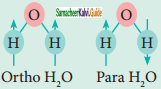At room temperature, normal hydrogen consists of about 75% ortho-form and 25% para-form. As the ortho-form is more stable than para-form, the conversion of one isomer into the other is a slow process. However, the equilibrium shifts in favour of para hydrogen when the temperature is lowered.

The para-form can be catalytically transformed into ortho-form using platinum or iron. Alternatively, it can also be converted by passing an electric discharge, heating above 800°C and mixing with paramagnetic molecules such as O2, NO, NO2 or with nascent/atomic hydrogen.Question 5.
Discuss the methods of preparation of hydrogen.
High purity hydrogen (> 99.9%) is obtained by the electrolysis of water containing traces of acid or alkali or the electrolysis of aqueous solution of sodium hydroxide or potassium hydroxide using a nickel anode and iron cathode. However, this process is not economical for large-scale production.
At Anode:
2OH → H2O + $$\frac{1}{2}$$O2 + 2e

At Cathode:
2H2O + 2e → 2OH + H2

Overall Reaction:
H2O → H2 + $$\frac{1}{2}$$O2
Hydrogen is conveniently prepared in laboratory by the reaction of metals, such as zinc, iron, tin with dilute acid.
Zn + 2HCl → ZnCl2 + H2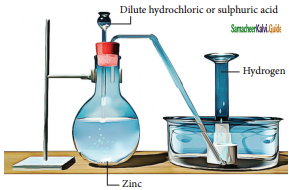Laboratory preparation of Hydrogen

Question 6.
Write the chemical properties of deuterium.
Like hydrogen, deuterium also reacts with oxygen to form deuterium oxide called heavy water. It also reacts with halogen to give corresponding halides.
2D2 + O2 → 2D2O
D2 + X2 → 2DX (X = F, Cl, Br & I)

Deuterium can replace reversibly hydrogen in compounds either partially or completely depending upon the reaction conditions. These reactions occur in the presence of deuterium or heavy water
CH4 + 2D2 → CD4 + 2H2
2NH3 + 3D2 → 2ND3 + 3H2

Question 7.
Write the reaction of halogens with water.
The halogens react with water to give an acidic solution. For example, chlorine forms hydrochloric acid and hypo chlorous acid. It is responsible for the antibacterial action of chlorine water, and for its use as bleach.
Cl2 + H2O → HCl + HOCl
Fluorine reacts differently to liberate oxygen from water.
2F2 + 2H2O → 4HF + O2

Question 8.
Discuss the nature of hydrated salts with suitable examples.
Many salts crystallized from aqueous solutions form hydrated crystals. The water in the hydrated salts may form co-ordinate bond or just present in interstitial positions of crystals.
Examples: Cr(H2O6) Cl3) – All six water molecules form co-ordinate bond. BaCl2.2H2O – Both the water molecules are present in interstitial positions.
CuSO4 .5H2O – In this compound four water molecules form co-ordinate bonds while the fifth water molecule, present outside the co-ordination, can form intermolecular hydrogen bond with another molecule. [Cu(H2O)4]SO4. H2O.Question 9.
How is temporary hardness of water removed by boiling?
Temporary hardness is primarily due to the presence of soluble bicarbonates of magnesium and calcium. This can be removed by boiling the hard water followed by filtration. Upon boiling, these salts decompose into insoluble carbonate which leads to their precipitation. The magnesium carbonate thus formed further hydrol used to give insoluble magnesium hydroxide.
Ca(HCO3)2 → CaCO3 + H2O + CO2Mg(HCO3) → MgCO3 + H2O
CO2MgCO3 + H2O → Mg(OH)2 + CO2
The resulting precipitates can be removed by filtration.

Question 10.
How is temporary hardness of water removed by Clark’s method?
In Clark’s method, calculated amount of lime is added to hard water containing the magnesium and calcium, and the resulting carbonates and hydroxides can be filtered-off.
Ca(HCO3)2 + Ca(OH)2 → 2CaCO3 + 2H2O
Mg(HCO3) + 2Ca(OH)2 → 2CaCO3+ Mg(OH)2 + 2H2O

Question 11.
Write the chemical properties of heavy water.
When compounds containing hydrogen are treated with D2O, hydrogen undergoes an exchange for deuterium 2NaOH + D2O → 2NaOD + HOD
HCl + D2O → DCl + HOD
NH4Cl + 4D2O → ND4Cl + 4HOD

These exchange reactions are useful in determining the number of ionic hydrogens present in a given compound. For example, when D2O is treated with of hypo-phosphorus acid only one hydrogen atom is exchanged with deuterium. It indicates that, it is a monobasic acid.
H3PO2 + D2O → H2DPO2 + HDO
It is also used to prepare some deuterium compounds:
Al4C3 + 12D2O → 4Al(OD)3 + 3CD4
CaC2 + 2D2O → Ca(OD)2 + C2D2
MgN2 + 6D2O → 3Mg(OD)2 + 2ND3
Ca3P2 + 6D2O → 3Ca(OD)2 + 2PD3Question 12.
Write the uses of heavy water.
(i) Heavy water is widely used as moderator in nuclear reactors as it can lower the energies of fast neutrons
(ii) It is commonly used as a tracer to study organic reaction mechanisms and mechanism of metabolic reactions
(iii) It is also used as a coolant in nuclear reactors as it absorbs the heat generated.

Question 13.
Write the uses of hydrogen peroxide.
The oxidizing ability of hydrogen peroxide and the harmless nature of its products, i.e., water and oxygen, lead to its many applications. It is used in water treatment to oxidize pollutants, as a mild antiseptic, and as bleach in textile, paper and hair – care industry.

Hydrogen peroxide is used to restore the white colour of the old paintings which was lost due to the reaction of hydrogen sulphide in air with the white pigment Pb3(OH)2 (CO3)2 to form black colored lead sulphide. Hydrogen peroxide oxidises black coloured lead sulphide to white coloured lead sulphate, there by restoring the colour.
PbS + 4H2O2 → PbSO4 + 4H2OIV. Long Question and Answers (5 Marks):

Question 1.
Justify the position of hydrogen in the periodic table.
The hydrogen has the electronic configuration of 1s1 which resembles with ns1 general valence shell configuration of alkali metals and shows similarity with them as follows:
(i) It forms unipositive ion (H+) like alkali metals (Na+, K+, Cs+)
(ii) It forms halides (HX), oxides (H2O), peroxides (H2O2) and sulphides (H2S) like alkali metals (NaX, Na2O, Na2O2, Na2S)
(iii) It also acts as a reducing agent.

However, unlike alkali metals which have ionization energy ranging from 377 to 520 kJ mol-1, the hydrogen has 1,314kJ mol-1 which is much higher than alkali metals.

Like the formation of halides (X) from halogens, hydrogen also has a tendency to gain one electron to form hydride ion (H) whose electronic configuration is similar to the noble gas, helium. However, the electron affinity of hydrogen is much less than that of halogen atoms. Hence, the tendency of hydrogen to form hydride ion is low compared to that of halogens to form the halide ions as evident from the following reactions:

$$\frac{1}{2}$$ H2 + e → H                                 ∆H = + 36 kcalmol-1
$$\frac{1}{2}$$ Br2 + e → Br                                 ∆H = -55 kcalmol-1

Since, hydrogen has similarities with alkali metals as well as the halogens; it is difficult to find the right position in the periodic table. However, in most of its compounds hydrogen exists in+1 oxidation state. Therefore, it is reasonable to place the hydrogen in group 1 along with alkali metals as shown in the latest periodic table published by IUPAC.

Question 2.
Discuss the reaction of hydrogen with (i) Oxygen (ii) Halogens (iii) Alkali metals.
(i) Reaction of hydrogen with oxygen.
Hydrogen reacts with oxygen to give water. This is an explosive reaction and releases lot of energy. This is used in fuel cells to generate electricity.
2H2 + O2 → 2H2O

(ii) Reaction of hydrogen with halogens.
Similarly, hydrogen also reacts with halogens to give corresponding halides. Reaction with fluorine takes place even in dark with explosive violence while with chlorine at room temperature under light. It combines with bromine on heating and reaction with iodine is a photochemical reaction.
2H2 + O2 → 2H2O
In the above reactions the hydrogen has an oxidation state of +1.

(iii) Reaction of hydrogen with alkali metals:
It also has a tendency to react with reactive metals such as lithium, sodium and calcium to give corresponding hydrides in which the oxidation state of hydrogen is -1.
2Li + H2 → 2 LiH
2Na + H2 → 2NaH

These hydrides are used as reducing agents in synthetic organic chemistry. It is used to prepare other important hydrides such as lithium aluminium hydride and sodium boro hydride.
4 LiH + AlCl3 → Li[AlH4] + 3LiCl
4 NaH + B(OCH3)3 → Na[BH4] + 3CH3ONaQuestion 3.
Explain the uses of hydrogen.
1. Over 90 % hydrogen produced in industry is used for synthetic applications. One such process is Haber process which is used to synthesis ammonia in large scales. Ammonia is used for the manufacture of chemicals such as nitric acid, fertilizers and explosives.
N2 + 3H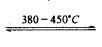2NH
2. It can be used to manufacture the industrial solvent, methanol from carbon monoxide using copper as catalyst.
CO + 2H2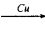CH3OH
3. Unsaturated fatty oils can be converted into saturated fats called Vanaspati (margarine) by the reduction reaction with $$\frac{P_{t}}{H_{2}}$$
4. In metallurgy, hydrogen can be used to reduce many metal oxides to metals at high temperatures.
CuO + H2 → Cu + H2O

WO3 + 3H2 → W + 3H2O
5. Atomic hydrogen and oxy-hydrogen torches are used for cutting and welding.
6. Liquid hydrogen is used as a rocket fuel.
7. Hydrogen is also used in fuel cells for generating electrical energy. The reversible uptake of hydrogen in metals is also attractive for rechargeable metal hydride battery.

Question 4.
What is permanent hardness of water? How is it removed?
Permanent hardness of water is due to the presence of soluble salts of magnesium and calcium in the form of chlorides and sulphates in it. It can be removed by adding washing soda, which reacts with these metal {M = Ca or Mg) chlorides and sulphates in hard water to form insoluble carbonates.

MCl2 + Na2CO3 → MCO3+ 2NaCl
MSO4 + Na2CO3 → MCO3 + Na2SO4

In another way to soften the hard water is by using a process called ion-exchange. That is, hardness can be removed by passing through an ion-exchange bed like zeolites or column containing ion-exchange resin. Zeolites are hydrated sodium alumino-silicates with a general formula, NaO . Al2O3 . xSiO2. yH2O (x = 2 to 10, y = 2 to 6).

Zeolites have porous structure in which the monovalent sodium ions are loosely held and can be exchanged with hardness producing metal ions (M= Ca or Mg) in water. The complex structure can conveniently be represented as Na2 – Z with sodium as exchangeable cations.
Na2 – Z + M2+ → M – Z + 2Na+

When exhausted, the materials can be regenerated by treating with aqueous sodium chloride. The metal ions (Ca2 and Mg2+) caught in the zeolite (or resin) are released and they get replenished with sodium ions.
M – Z + 2NaCl → Na2 – Z + MCl2Question 5.
Write the chemical properties and uses of heavy water.
When compounds containing hydrogen are treated with D2O, hydrogen undergoes an exchange for deuterium 2NaOH + D2O → 2NaOD + HOD
HCl + D2O → DCl + HOD
NH4Cl + 4D2O → ND4Cl + 4HOD

These exchange reactions are useful in determining the number of ionic hydrogens present in a given compound. For example, when D2O is treated with of hypo-phosphoric acid only one hydrogen atom is exchanged with deuterium. It indicates that, it is a monobasic acid.
H3PO2 +D2O → H2DPO2 + HDO

It is also used to prepare some deuterium compounds:
Al4Cl3 + 12D2O → 4Al(OD)3 + 3CD4
CaC2 + 2D2O → Ca(OD)2 + C2D2
Mg3N2 + 6D2O → 3Mg(OD)2 + 2ND3
Ca3P2 + 6D2O → 3Ca(OD)2 + 2PD3

Uses of Heavy Water:
1. Heavy water is widely used as moderator innuclear reactors as it can lower the energies of fast neutrons
2. It is commonly used as a tracer to study organic reaction mechanisms and mechanism of metabolic reactions
3. It is also used as a coolant in nuclear reactors as it absorbs the heat generated.

Question 6.
Explain the structure of hydrogen peroxide.
Both in gas-phase and liquid-phase, the molecule adopts a skew conformation due to repulsive interaction of the OH bonds with lone-pairs of electrons on each oxygen atom. Indeed, it is the smallest molecule known to show hindered rotation • about a single bond.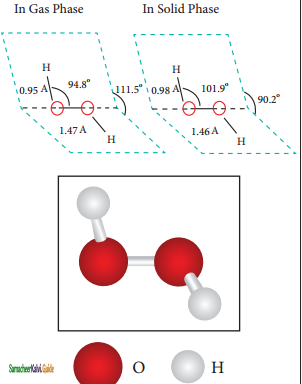H2O2 has a non-polar structure. The molecular dimensions in the gas phase and solid phase differ as shown in figure 4.5. Structurally, H2O2 is represented by the dihydroxyl formula in which the two OH-groups do not lie in the same plane.

One way of explaining the shape of hydrogen peroxide is that the hydrogen atoms would lie on the pages of a partly opened book, and the oxygen atoms along the spine. In the solid phase of molecule, the dihedral angle reduces to 90.2° due to hydrogen bonding and the O-O-H angle expands from 94.8° to 101.9°.Question 7.
What are hydrides? How are they classified?
Hydrogen forms binary hydrides with many electropositive elements including metals and non-metals. It also forms ternary hydrides with two metals. E.g., LiBH4 and LiAlH4 The hydrides are classified as ionic, covalent and metallic hydrides according to the nature of bonding.

Hydrides formed with elements having lower electronegativity than hydrogen are often ionic, whereas with elements having higher electronegativity than hydrogen form covalent hydrides.

Ionic (Saline) hydrides:
These are hydrides composed of an electropositive metal, generally, an alkali or alkaline-earth metal, except beryllium and magnesium, formed by transfer of electrons from metal to hydrogen atoms. They can be prepared by the reaction of elements at about 400° These are salt-like, high-melting, white crystalline solids having hydride ions (H) and metal cations (Mn+).
2 Li + H2 → 2LiH

Covalent (Molecular) hydrides:
They are compounds in which hydrogen is attached to another element by sharing of electrons. The most common examples of covalent hydrides of non-metals are methane, ammonia, water and hydrogen chloride. Covalent hydrides are further divided into three categories, viz., electron precise (CH4, C2H6, SiH4, GeH4), electron-deficient(B2H6) and electron-rich hydrides (NH3, H2O). Since most of the covalent hydrides consist of discrete, small molecules that have relatively weak intermolecular forces, they are generally gases or volatile liquids.

Metallic (Interstitial) hydrides:
Metallic hydrides are usually obtained by hydrogenation of metals and alloys in which hydrogen occupies the interstitial sites (voids). Hence, they are called interstitial hydrides; the hydrides show properties similar to parent metals and hence they are also known as metallic hydrides.

Most of the hydrides are non-stoichiometric with variable composition (TiH1.5 – 1.8 and PdH0.6 – 0.8), some are relatively light, inexpensive and thermally unstable which make them useful for hydrogen storage applications. Electropositive metals and some other metals form hydrides with the stoichiometry MH or sometimes MH2 (M = Ti, Zr, Hf, V, Zn).

Question 8.
Write notes on intermolecular hydrogen bonding.
Intermolecular hydrogen bonds occur between two separate molecules. They can occur between any numbers of like or unlike molecules as long as hydrogen donors and acceptors are present in positions which enable the hydrogen bonding interactions. For example, intermolecular hydrogen bonds can occur between ammonia molecule themselves or between water molecules themselves or between ammonia and water.

Water molecules form strong hydrogen bonds with one another. For example, each water molecule is linked to four others through hydrogen bonds. The shorter distances (100 pm) correspond to covalent bonds (solid lines), and the longer distances (180 pm) correspond to hydrogen bonds (dotted lines).

In ice, each atom is surrounded tetrahedrally by four water molecules through hydrogen bonds. That is, the presence of two hydrogen atoms and two lone pairs of electron on oxygen atoms in each water molecule allows formation of a three-dimensional structure. This arrangement creates an open structure, which accounts for the lower density of ice compared with water at 0°C. While in liquid water, unlike ice where hydrogen bonding occurs over a long-range, the strong hydrogen bonding prevails only in a short range and therefore the denser packing.Question 9.
What is hydrogen bonding? Discuss its properties and applications.